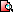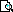Basics Archives Community Services ProgrammingHome :: Archives :: File Archives :: Authors :: Edmond WongEdmond Wong

AUTHOR PROFILE

Ranked number 536 in authors with the most files with 12 files.

FILES BY THIS AUTHOR

Related Files
File Title Categoryambgcase.zip The Ambiguous Case TI-83/84 Plus BASIC Math Programs (Geometry)distform.zip Distance Formula TI-83/84 Plus BASIC Math Programs (Geometry)heron.zip Heron's Formula TI-83/84 Plus BASIC Math Programs (Geometry)midpointformula.zip Midpoint Formula. TI-83/84 Plus BASIC Math Programs (Geometry)numgame.zip Number Guessing Game. TI-83/84 Plus BASIC Games (Chance/Guessing)pyth.zip Edmond's Pythagorean Theorem TI-83/84 Plus BASIC Math Programs (Geometry)quad4.zip Edmond's Quadratic Formula TI-83/84 Plus BASIC Math Programs (Quadratic Solvers)quadformula.zip Quadratic Equation Solver TI-83/84 Plus BASIC Math Programs (Quadratic Solvers)slopeform.zip Slope Formula. TI-83/84 Plus BASIC Math Programs (Algebra)svmethod.zip Surveyor's Method. TI-83/84 Plus BASIC Math Programs (Geometry)traparea.zip Trapezoid Area. TI-83/84 Plus BASIC Math Programs (Geometry)triareaform.zip Triangle Area. TI-83/84 Plus BASIC Math Programs (Geometry)